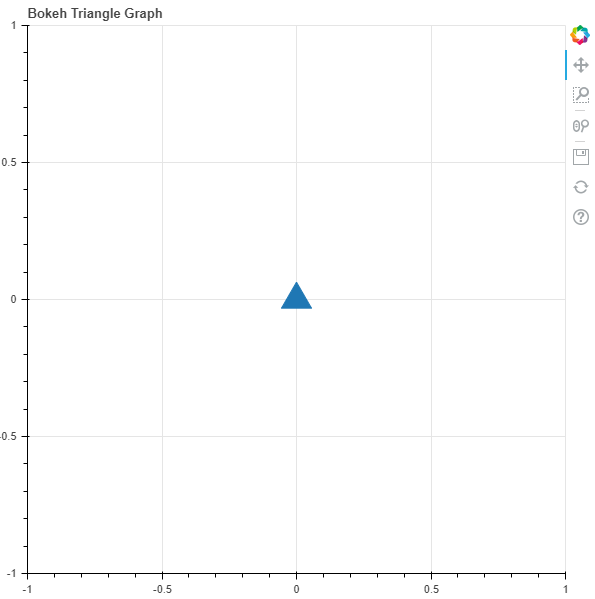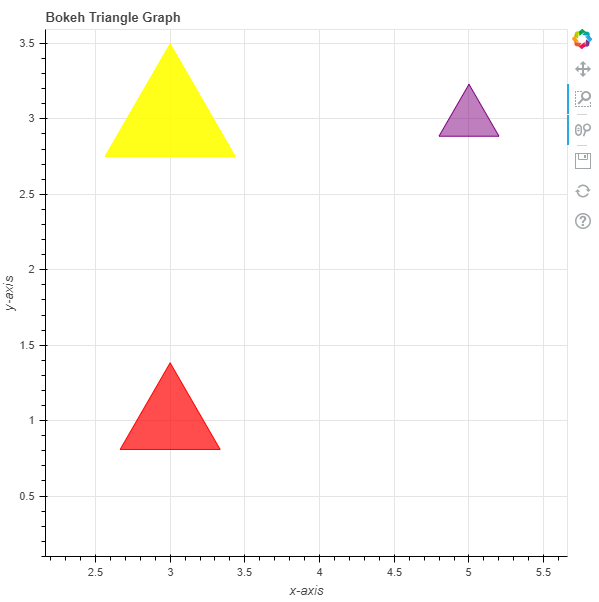# Python Bokeh – Plotting Triangles on a Graph

• Last Updated : 10 Jul, 2020

Bokeh is a Python interactive data visualization. It renders its plots using HTML and JavaScript. It targets modern web browsers for presentation providing elegant, concise construction of novel graphics with high-performance interactivity.

Bokeh can be used to plot triangles on a graph. Plotting triangles on a graph can be done using the `triangle()` method of the `plotting` module.

## plotting.figure.triangle()

Syntax : triangle(parameters)

Parameters :

• x : x-coordinates of the center of the triangle markers
• y : y-coordinates of the center of the triangle markers

Returns : an object of class `GlyphRenderer`

Example 1 : In this example we will be using the default values for plotting the graph.

 `# importing the modules``from` `bokeh.plotting ``import` `figure, output_file, show``        ` `# file to save the model``output_file(``"gfg.html"``)``        ` `# instantiating the figure object``graph ``=` `figure(title ``=` `"Bokeh Triangle Graph"``)``      ` `# the points to be plotted``x ``=` `0``y ``=` `0``     ` `# plotting the graph``graph.triangle(x, y, size ``=` `30``)``      ` `# displaying the model``show(graph) `

Output :Example 2 : In this example we will be plotting multiple triangles with various other parameters.

 `# importing the modules ``from` `bokeh.plotting ``import` `figure, output_file, show ``  ` `# file to save the model ``output_file(``"gfg.html"``) ``       ` `# instantiating the figure object ``graph ``=` `figure(title ``=` `"Bokeh Triangle Graph"``) ``  ` `# name of the x-axis ``graph.xaxis.axis_label ``=` `"x-axis"``       ` `# name of the y-axis ``graph.yaxis.axis_label ``=` `"y-axis"``  ` `# points to be plotted``x ``=` `[``3``, ``3``, ``5``]``y ``=` `[``3``, ``1``, ``3``]``size ``=` `[``130``, ``100``, ``60``]``  ` `# color value of the triangles``color ``=` `[``"yellow"``, ``"red"``, ``"purple"``]``  ` `# fill alpha value of the triangles``fill_alpha ``=` `[``0.9``, ``0.7``, ``0.5``]``  ` `# plotting the graph ``graph.triangle(x, y,``               ``size ``=` `size,``               ``color ``=` `color,``               ``fill_alpha ``=` `fill_alpha) ``       ` `# displaying the model ``show(graph)`

Output :My Personal Notes arrow_drop_up